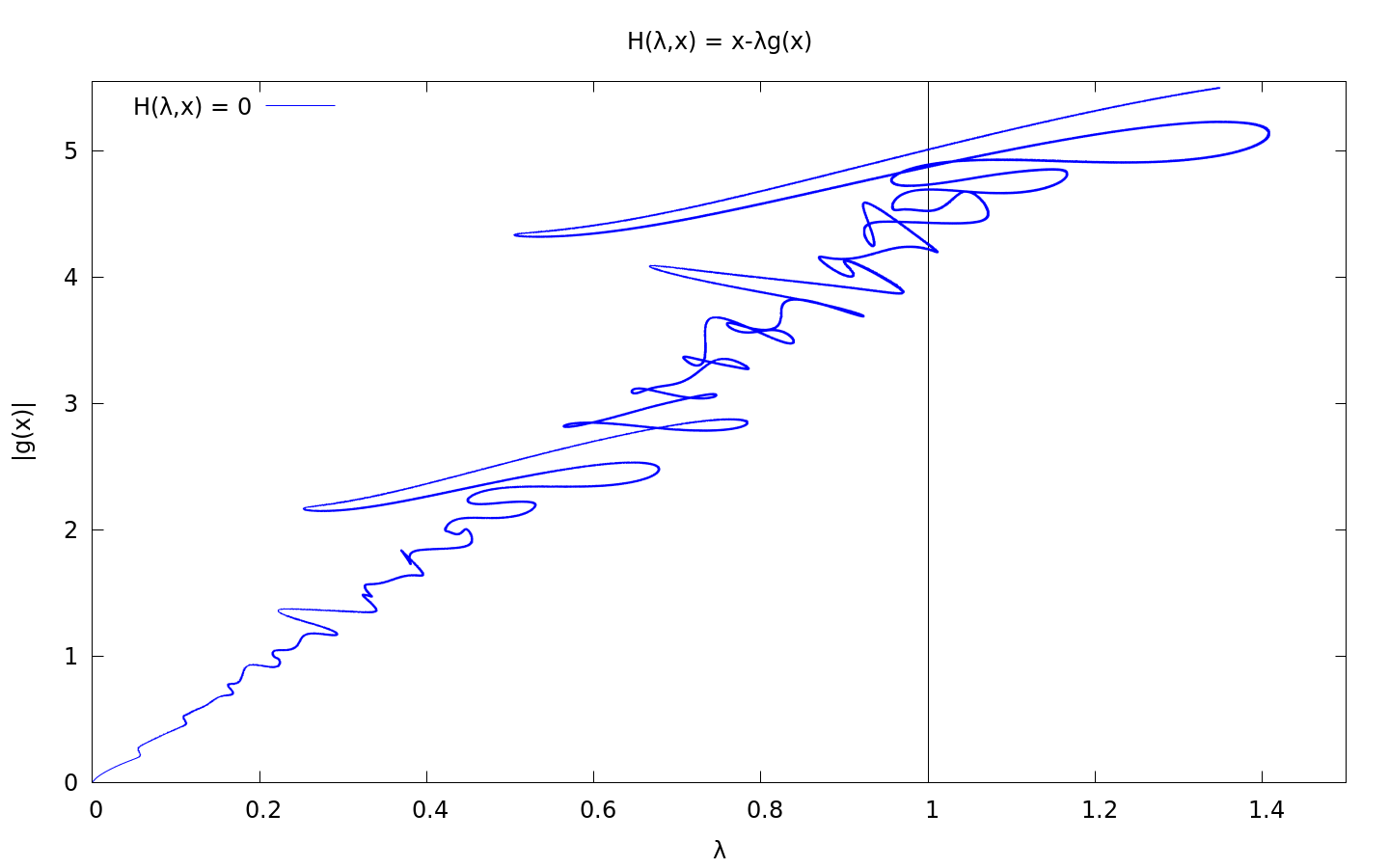## Traceu

Piecewise-linear tracing utility for implicitly defined curves## Example: Fixpoints

Let $$g: \mathbb{R}^n \rightarrow \mathbb{R}^n, g_k(x) = \exp\Big( \cos\Big( k \cdot \sum\limits_{i=1}^n x_i \Big)\Big)$$ for $$k = 1,\ldots,n$$

Considering the homotopy

$$H:[0,1] \times \mathbb{R}^n \rightarrow \mathbb{R}^n, (\lambda,x) \mapsto x - \lambda g(x)$$

it is obvious, that $$H(0,x) = x$$ and $$H(1,x) = 0 \Leftrightarrow x = g(x)$$ One approach to find fixpoints of $$g$$ is therefore to follow $$H^{-1}(0)$$ starting at $$x=0$$ until $$\lambda = 1$$ holds. traceu provides piecewise linear approximations of such curves with upper bounds for the error enabling further (e.g. newton-type) methods to be successfully applied on the results. The picture above depicts a trace of $$H^{-1}(0)$$ for $$n=10$$.